# ELECTRIC CURRENTS WHAT IS ELECTRIC CURRENT An electric

• Slides: 14ELECTRIC CURRENTS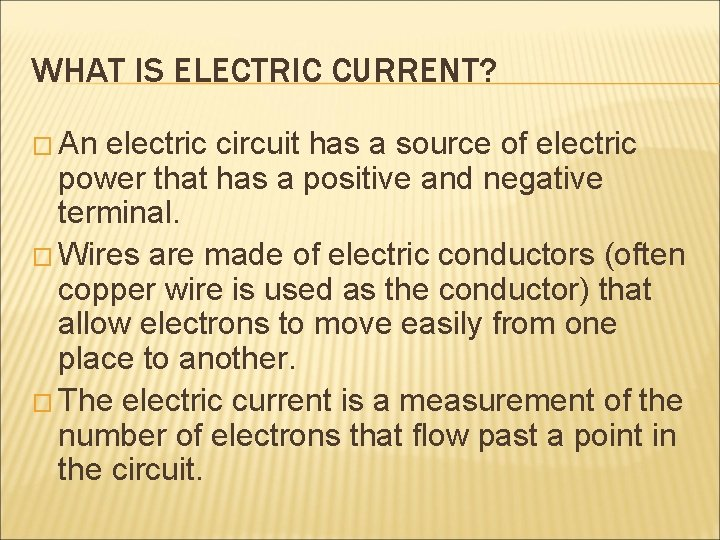WHAT IS ELECTRIC CURRENT? � An electric circuit has a source of electric power that has a positive and negative terminal. � Wires are made of electric conductors (often copper wire is used as the conductor) that allow electrons to move easily from one place to another. � The electric current is a measurement of the number of electrons that flow past a point in the circuit.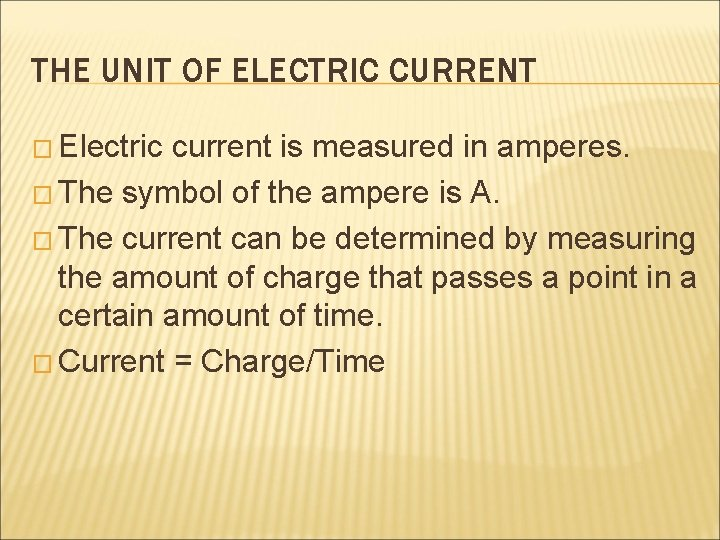THE UNIT OF ELECTRIC CURRENT � Electric current is measured in amperes. � The symbol of the ampere is A. � The current can be determined by measuring the amount of charge that passes a point in a certain amount of time. � Current = Charge/TimeTYPES OF ELECTRIC CURRENT � There are two different types of electric current: � Direct Current (DC) � Alternating Current (AC)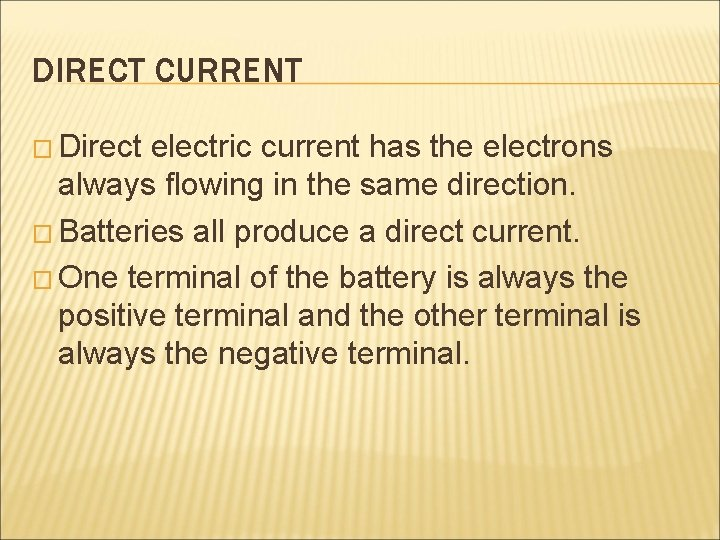DIRECT CURRENT � Direct electric current has the electrons always flowing in the same direction. � Batteries all produce a direct current. � One terminal of the battery is always the positive terminal and the other terminal is always the negative terminal.ALTERNATING CURRENT � Alternating current has the electrons flowing in one direction at one time and then the current flow is reversed and the electrons move in the opposite direction. � Generators usually provide alternating current. � There are ways to convert from one form of current to another.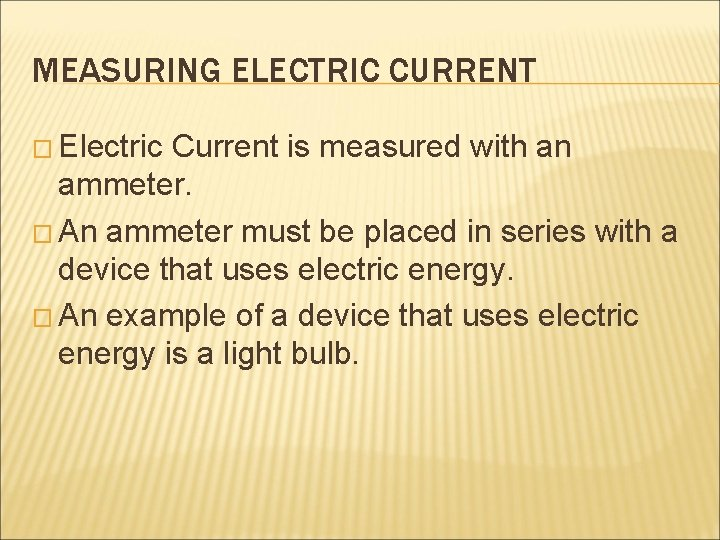MEASURING ELECTRIC CURRENT � Electric Current is measured with an ammeter. � An ammeter must be placed in series with a device that uses electric energy. � An example of a device that uses electric energy is a light bulb.DIRECTION OF ELECTRIC CURRENT � Electric current was measured before scientists understood that it was the negatively charged electrons that move the current around an electric circuit. � Conventional Current in a DC circuit is shown going from the positive terminal to the negative terminal of a battery or power source. It is represented by the letter I. � Electron Flow in a DC circuit shows the direction of the electrons flowing. It is represented by e-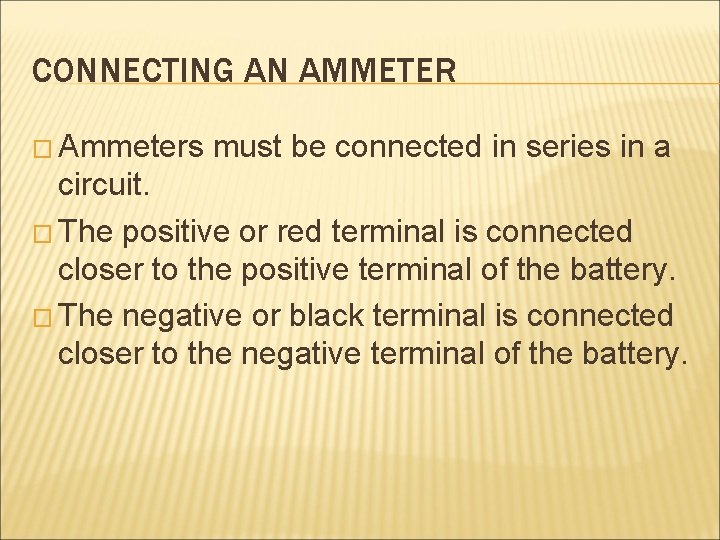CONNECTING AN AMMETER � Ammeters must be connected in series in a circuit. � The positive or red terminal is connected closer to the positive terminal of the battery. � The negative or black terminal is connected closer to the negative terminal of the battery.Digital Ammeter Negative Terminal Positive Terminal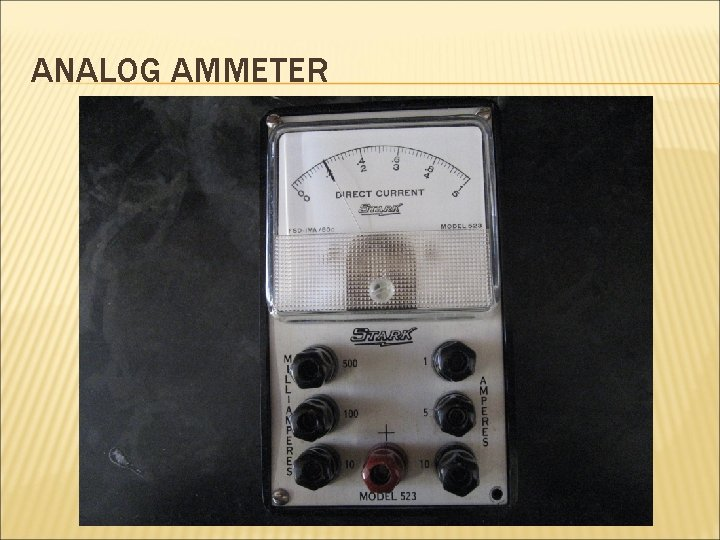ANALOG AMMETERANALOG AMMETERSelecting Switch Positive Terminal for Current Negative Terminal